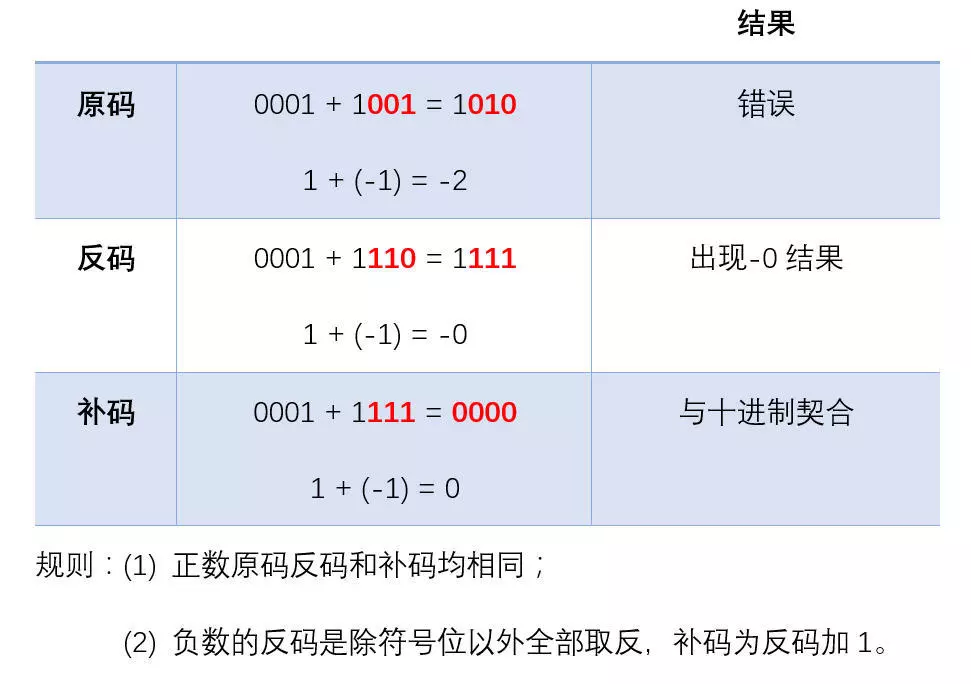184°

# Java中的Comparable接口和Comparator接口# 1. 介绍

`Comparable<T>`接口和`Comparator<T>`接口都是JDK中提供的和比较相关的接口。使用它们可以对对象进行比较大小，排序等操作。这算是之后排序的先导知识吧。
`Comparable`， 字面意思是“可以比较的”，所以实现它的类的多个实例应该可以相互比较“大小”或者“高低”等等。
`Comparator`， 字面意思是“比较仪，比较器”， 它应该是专门用来比较用的“工具”。

# 2. Comparable

`Comparable<T>`接口

``````public interface Comparable<T> {

public int compareTo(T o);
}
``````

This interface imposes a total ordering on the objects of each class that implements it. This ordering is referred to as the class's <i>natural ordering</i>, and the class's <tt>compareTo</tt> method is referred to as its <i>natural comparison method</i>.<p>

Lists (and arrays) of objects that implement this interface can be sorted automatically by {@link Collections#sort(List) Collections.sort} (and {@link Arrays#sort(Object[]) Arrays.sort}).

``````public final class Integer extends Number implements Comparable<Integer> {

private final int value;

public int compareTo(Integer anotherInteger) {
return compare(this.value, anotherInteger.value);
}
public static int compare(int x, int y) {
return (x < y) ? -1 : ((x == y) ? 0 : 1);
}
public static int compareUnsigned(int x, int y) {
return compare(x + MIN_VALUE, y + MIN_VALUE);
}
``````

``````    public static int compareUnsigned(int x, int y) {
return compare(x + MIN_VALUE, y + MIN_VALUE);
}
``````

## 2.1 计算机编码

`1`的二进制为`0001``-1`的二进制为`1001`，如果直接相加，则`1 + （-1） = 0`，二进制表示为`0001 + 1001 = 1010 != 0`，所以不能直接使用原码做运算。

`(-1) + (-7) = (补码) 1111 + 1001 = 1000 = -8`。注意，由于此处的`-8`使用了之前`-0`的补码来表示，所以`-8`没有没有原码和反码表示（针对的四位，如果是八位，则没有原码和反码的是`-128`，依次类推）。## 2.2 大数溢出问题

`int`类型在32位系统中占4个字节、32bit，补码表示的的数据范围为：

[10000000 00000000 00000000 00000000] ~ [01111111 11111111 11111111 11111111]

[−231,231−1]
[-2147483648, 2147483647]

[Integer.MIN_VALUE, Integer.MAX_VALUE]

`byte`类型的表示一样，由于负数比正数多表示了一个数字。对下限去相反数后的数值会超过上限值，溢出到下限，因此下限的相反数与下限相等；对上限去相反数的数值为负值，该负值比下限的负值大1，在可以表示的范围内，因此上限的相反数是上限直接取负值。

## 2.3 String类型的compareTo方法

``````public final class String
implements java.io.Serializable, Comparable<String>, CharSequence {
/** The value is used for character storage. */
private final char value[];     // String的值

public int compareTo(String anotherString) {
int len1 = value.length;
int len2 = anotherString.value.length;
int lim = Math.min(len1, len2);     // limit， 表示两个String中长度较小的String长度
char v1[] = value;
char v2[] = anotherString.value;

int k = 0;
while (k < lim) {
char c1 = v1[k];
char c2 = v2[k];
if (c1 != c2) {
return c1 - c2;     // 如果char不相同，则取其差值
}
k++;    // 如果char值相同，则继续往后比较
}
return len1 - len2;     // 如果所有0 ~ (lim - 1)的char均相同，则比较两个String的长短
}
// 字面意思是对大小写不敏感的比较器
public static final Comparator<String> CASE_INSENSITIVE_ORDER
= new CaseInsensitiveComparator();
private static class CaseInsensitiveComparator
implements Comparator<String>, java.io.Serializable {
private static final long serialVersionUID = 8575799808933029326L;

public int compare(String s1, String s2) {
int n1 = s1.length();
int n2 = s2.length();
int min = Math.min(n1, n2);     // 和上面类似，均是取两个String间的最短长度
for (int i = 0; i < min; i++) {
char c1 = s1.charAt(i);
char c2 = s2.charAt(i);
if (c1 != c2) {
c1 = Character.toUpperCase(c1); // 统一换成大写
c2 = Character.toUpperCase(c2); // 统一换成大写
if (c1 != c2) {     // 大写如果不相等则再换为小写试试
c1 = Character.toLowerCase(c1);
c2 = Character.toLowerCase(c2);
if (c1 != c2) {     // 到此处则确定不相等
// No overflow because of numeric promotion
return c1 - c2;
}
}
}
}
return n1 - n2;
}

/** Replaces the de-serialized object. */
private Object readResolve() { return CASE_INSENSITIVE_ORDER; }
}
// String的方法，可以直接使用这个方法和其它String进行比较，
// 内部实现是调用内部比较器的compare方法
public int compareToIgnoreCase(String str) {
return CASE_INSENSITIVE_ORDER.compare(this, str);
}
}
``````

`String`中的关于`compare`的方法相对复杂一点，但还是比较简单。我们先不看其他的代码，只重点关注`compareTo`方法。

``````    public int compareTo(String anotherString) {
int len1 = value.length;
int len2 = anotherString.value.length;
int lim = Math.min(len1, len2);     // limit， 表示两个String中长度较小的String长度
char v1[] = value;
char v2[] = anotherString.value;

int k = 0;
while (k < lim) {
char c1 = v1[k];
char c2 = v2[k];
if (c1 != c2) {
return c1 - c2;     // 如果char不相同，则取其差值
}
k++;    // 如果char值相同，则继续往后比较
}
return len1 - len2;     // 如果所有0 ~ (lim - 1)的char均相同，则比较两个String的长短
}
``````

# 3. Comparator

`Comparator<T>`接口

``````public interface Comparator<T> {
int compare(T o1, T o2);
}
``````

`String`类中以及`Array`类等都有实现此接口的内部类。

``````    public static final Comparator<String> CASE_INSENSITIVE_ORDER
= new CaseInsensitiveComparator();
private static class CaseInsensitiveComparator
implements Comparator<String>, java.io.Serializable {
private static final long serialVersionUID = 8575799808933029326L;

public int compare(String s1, String s2) {
int n1 = s1.length();
int n2 = s2.length();
int min = Math.min(n1, n2);     // 和上面类似，均是取两个String间的最短长度
for (int i = 0; i < min; i++) {
char c1 = s1.charAt(i);
char c2 = s2.charAt(i);
if (c1 != c2) {
c1 = Character.toUpperCase(c1); // 统一换成大写
c2 = Character.toUpperCase(c2); // 统一换成大写
if (c1 != c2) {     // 大写如果不相等则再换为小写试试
c1 = Character.toLowerCase(c1);
c2 = Character.toLowerCase(c2);
if (c1 != c2) {     // 到此处则确定不相等
// No overflow because of numeric promotion
return c1 - c2;
}
}
}
}
return n1 - n2;
}

/** Replaces the de-serialized object. */
private Object readResolve() { return CASE_INSENSITIVE_ORDER; }
}
// String的方法，可以直接使用这个方法和其它String进行比较，
// 内部实现是调用内部比较器的compare方法
public int compareToIgnoreCase(String str) {
return CASE_INSENSITIVE_ORDER.compare(this, str);
}
}
``````

`CaseInsensitiveComparator`, 字面意思是对大小写不敏感的比较器。

1、具有工作经验的，面对目前流行的技术不知从何下手，需要突破技术瓶颈的可以加。

2、在公司待久了，过得很安逸，但跳槽时面试碰壁。需要在短时间内进修、跳槽拿高薪的可以加。

3、如果没有工作经验，但基础非常扎实，对java工作机制，常用设计思想，常用java开发框架掌握熟练的，可以加。

4、觉得自己很牛B，一般需求都能搞定。但是所学的知识点没有系统化，很难在技术领域继续突破的可以加。

5.阿里Java高级大牛直播讲解知识点，分享知识，多年工作经验的梳理和总结，带着大家全面、科学地建立自己的技术体系和技术认知！

我有话说: